• # Ways of thinking for mathematical research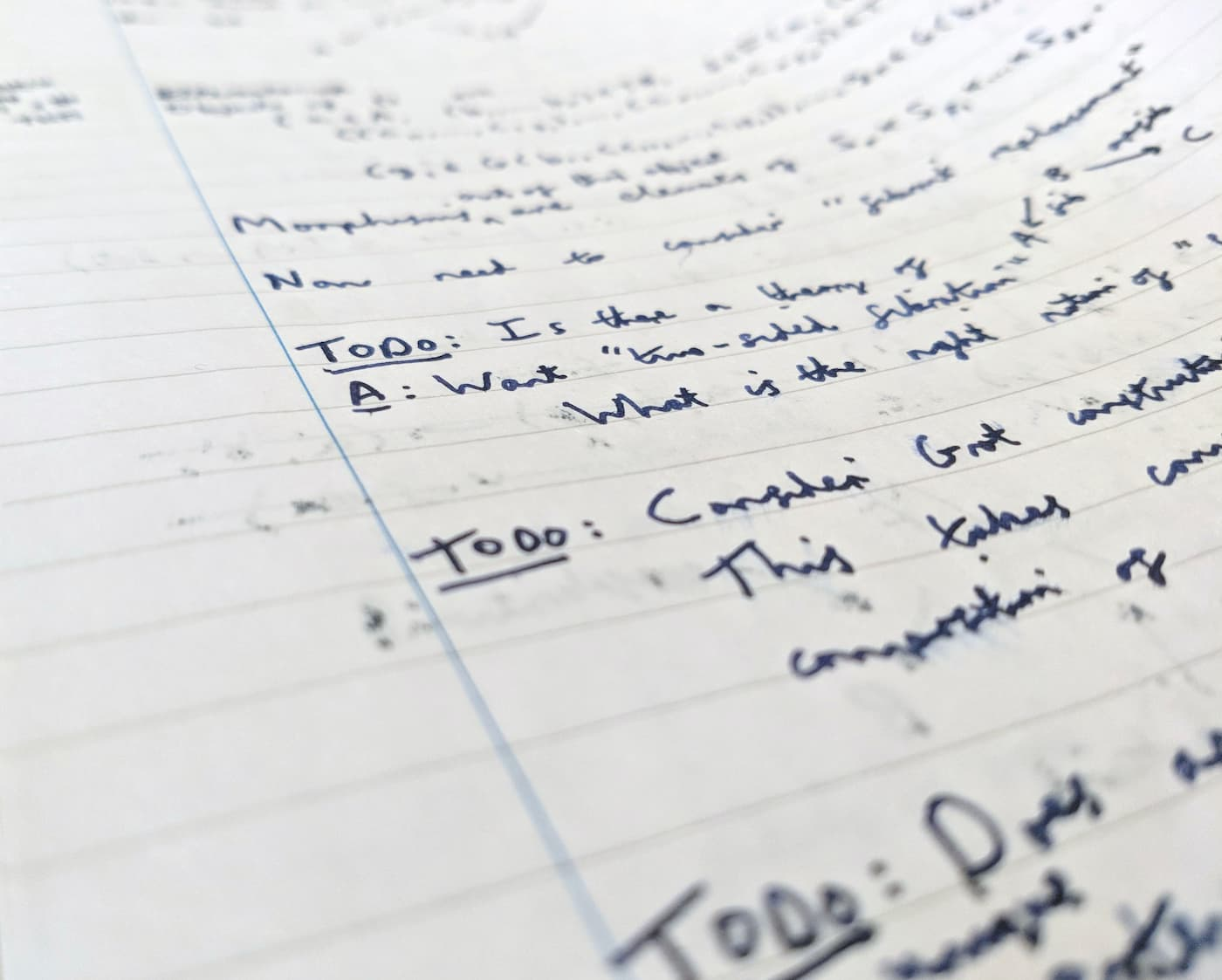Last summer I did an eight week mathematics research project, as mentioned in my previous post. This was my first real taste of mathematical research, and so I tried to write up some of my thoughts on lessons learned while they were still fresh in my mind. I just recently got around to editing this into a publishable form.

I’m sure very little of this is new to those of you who have experience in research – but I find it hard to remember what was difficult once I’m very used to something, so I hope there’s value in me writing this.

• # Slides on algebraic theories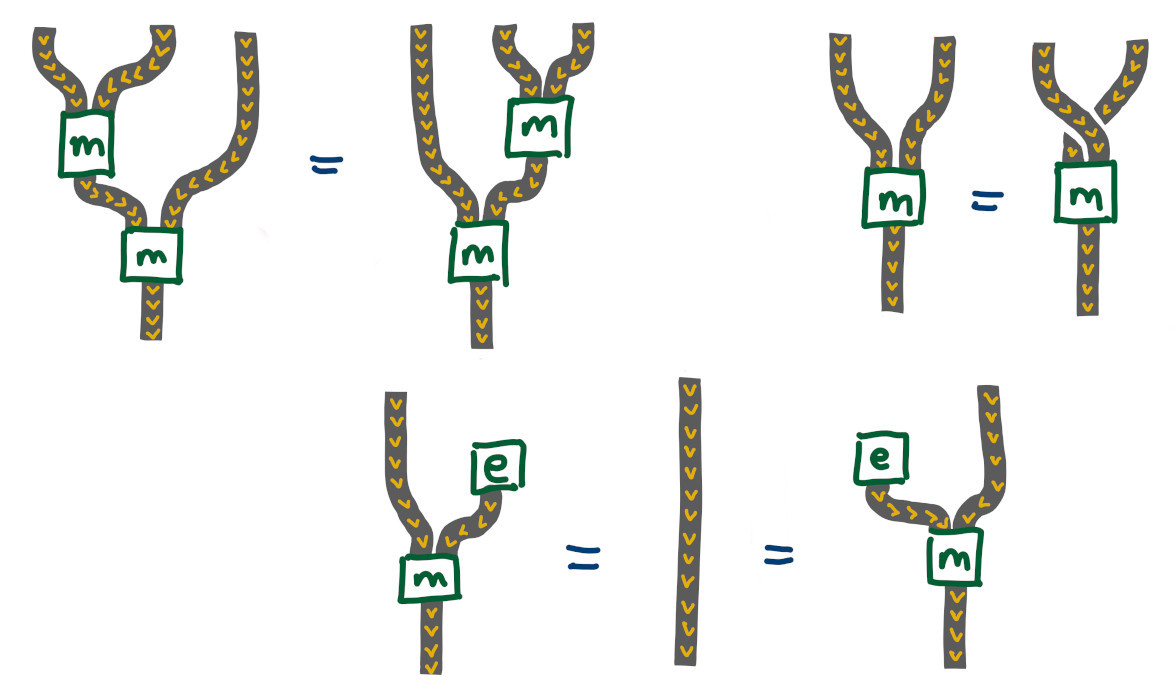I spent a couple months this summer doing a very enjoyable research project supervised by Martin Hyland, looking at several topics to do with algebraic theories. It was a great opportunity to get some idea of what research can be like, and to learn from someone with much more experience.

I recently gave a short talk about the project, and I’ve uploaded the slides. The talk was aimed at mathematics students who don’t know any category theory, so rather than taking a category-heavy point of view, I instead spent the first half explaining equational theories in some detail, while in the second half I tried to give a more intuitive description of the problems that I was actually working on.

• # What is a quasi-category?

A category describes a collection of objects with composable morphisms between them. In a 2-category you also have 2-morphisms between morphisms with matching domain and codomain. For example there is a 2-category $$\text{Cat}$$ with objects given by (small) categories, 1-morphisms given by functors between categories, and 2-morphisms given by natural transformations between functors. Continuing this pattern gives the idea of an $$n$$-category, where you have objects, 1-morphisms, 2-morphisms, and so on up to $$n$$-morphisms. The ultimate such structure is an $$\infty$$-category, with a never ending sequence of morphisms between morphisms between … between morphisms.

In a weak $$n$$- or $$\infty$$-category, associativity and identity laws only hold up to (coherent) isomorphism. In fact we don’t even need to require composition of morphisms to be uniquely defined! We only ask that all possible composites are uniquely isomorphic (where uniquely is interpreted in an appropriate higher categorical sense).

Weak higher categories are generally hard to understand, or even define correctly, and are currently an area of active research. However an especially well-behaved special case is that of a weak $$(\infty, 1)$$-category, where all $$k$$-morphisms for $$k > 1$$ are equivalences. Quasi-categories are one of the earliest and most important ways to formalise the idea of a weak $$(\infty, 1)$$-category. The definition of a quasi-category turns out to be less horrendously complicated than you might expect! The key is to understand simplicial sets, which are a kind of combinatorial approach to shape. Then a quasi-category is a simplicial set satisfying a condition that asks for certain “compositions of morphisms” to exist. We’ll start with a quick overview of simplicial sets, emphasising geometric intuition, before explaining the precise definition of a quasi-category.

## Simplicial sets

The idea of a simplicial set is to describe a shape by building it up out of simplices – points, lines, triangles, and so on. Moreover each simplex should come with an ordering on its vertices, so for example edges have a direction and triangles have an orientation.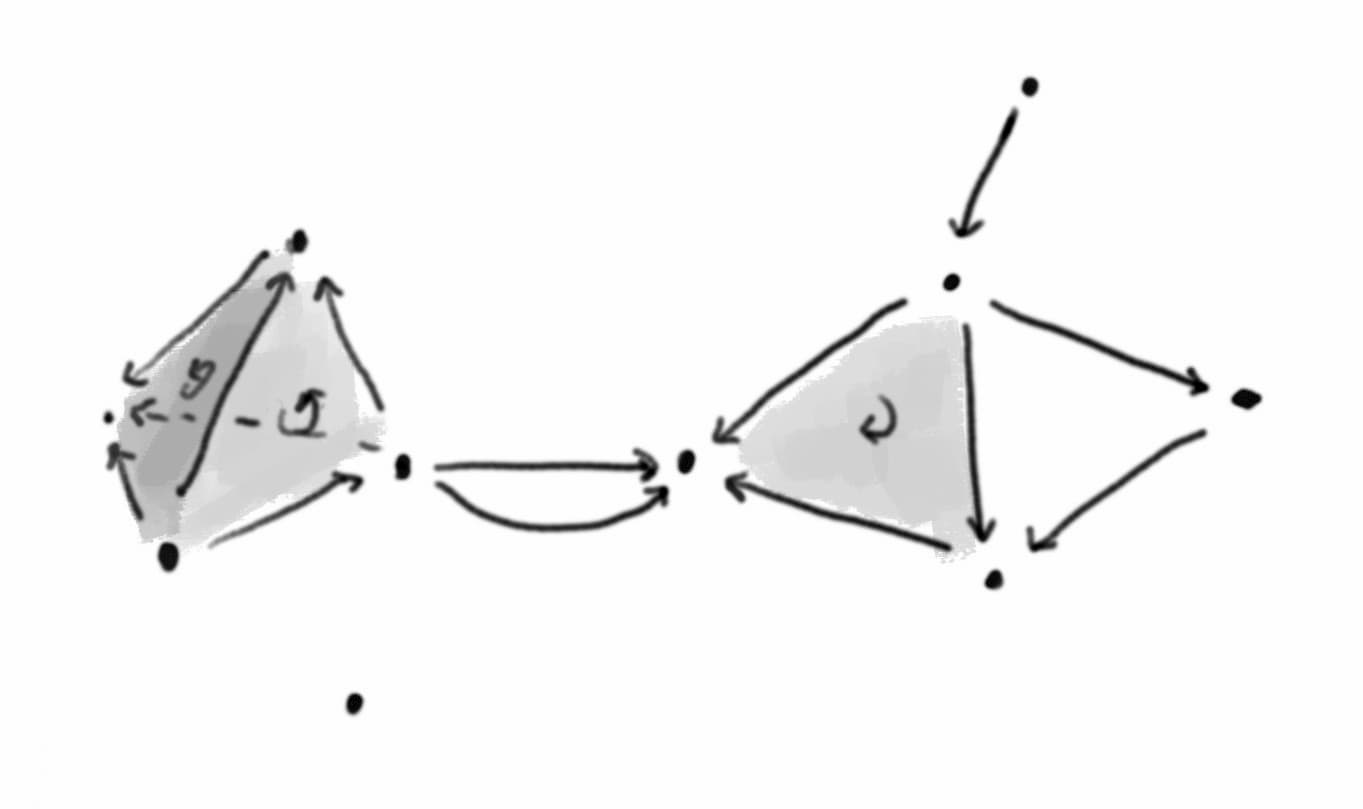• # Creative perspective

Tags: Art, Perspective

I’ve just started reading Ernest W. Watson’s Creative Perspective for Artists and Illustrators. I found that it cleared up several confusions I had about how perspective works, why correct perspective can seem distorted, and when and in what ways you might want to bend the rules.

I’ll follow Watson’s convention and refer to scientific perspective when I want to emphasise the idea of sticking precisely to the standard rules.

## What is perspective?

Stand in front of a window, and (keeping your head still and closing one eye) use a marker to trace what you see on the window. Then the resulting 2-dimensional picture will be in scientific perspective. The resulting image will match the image captured by an idealised camera.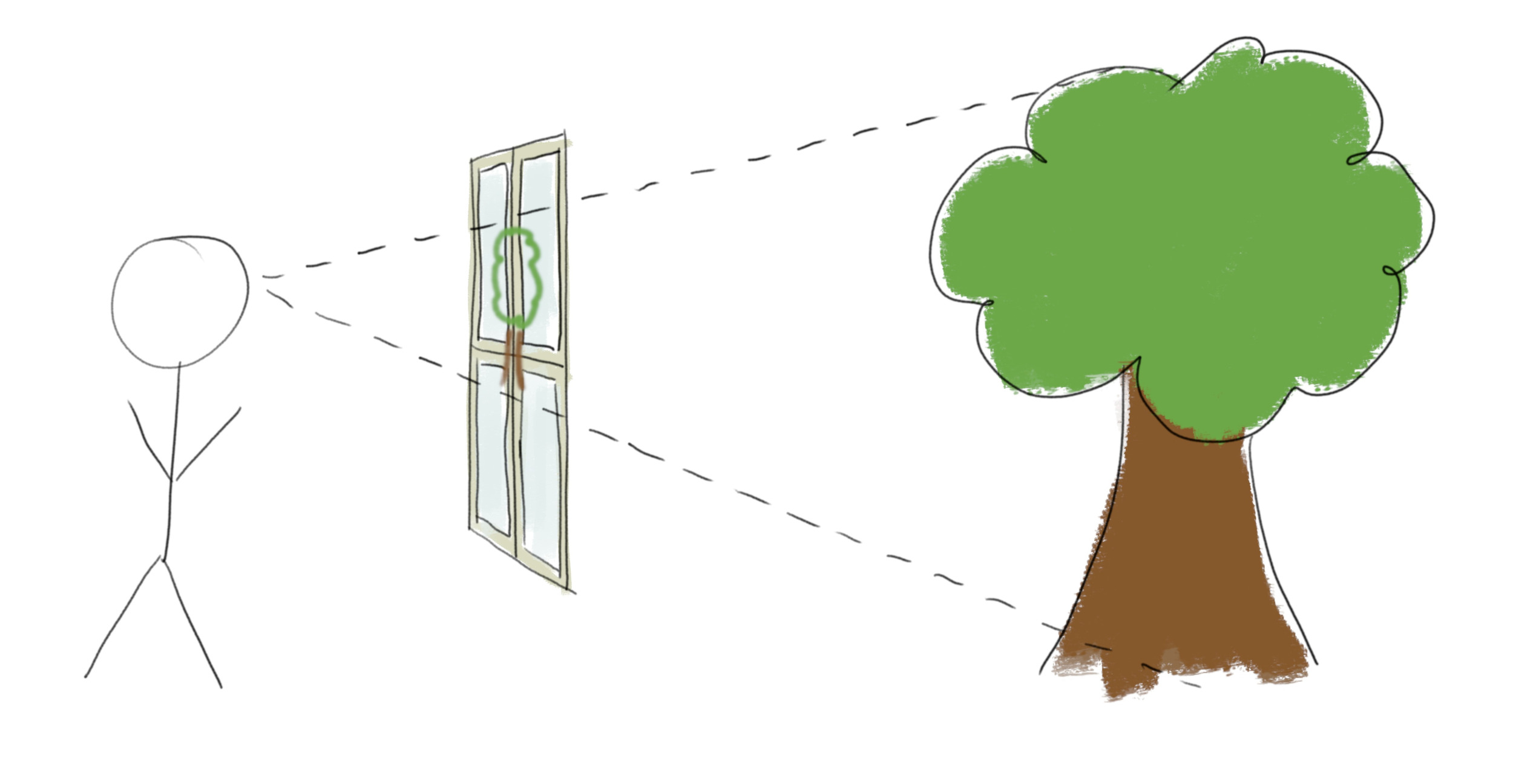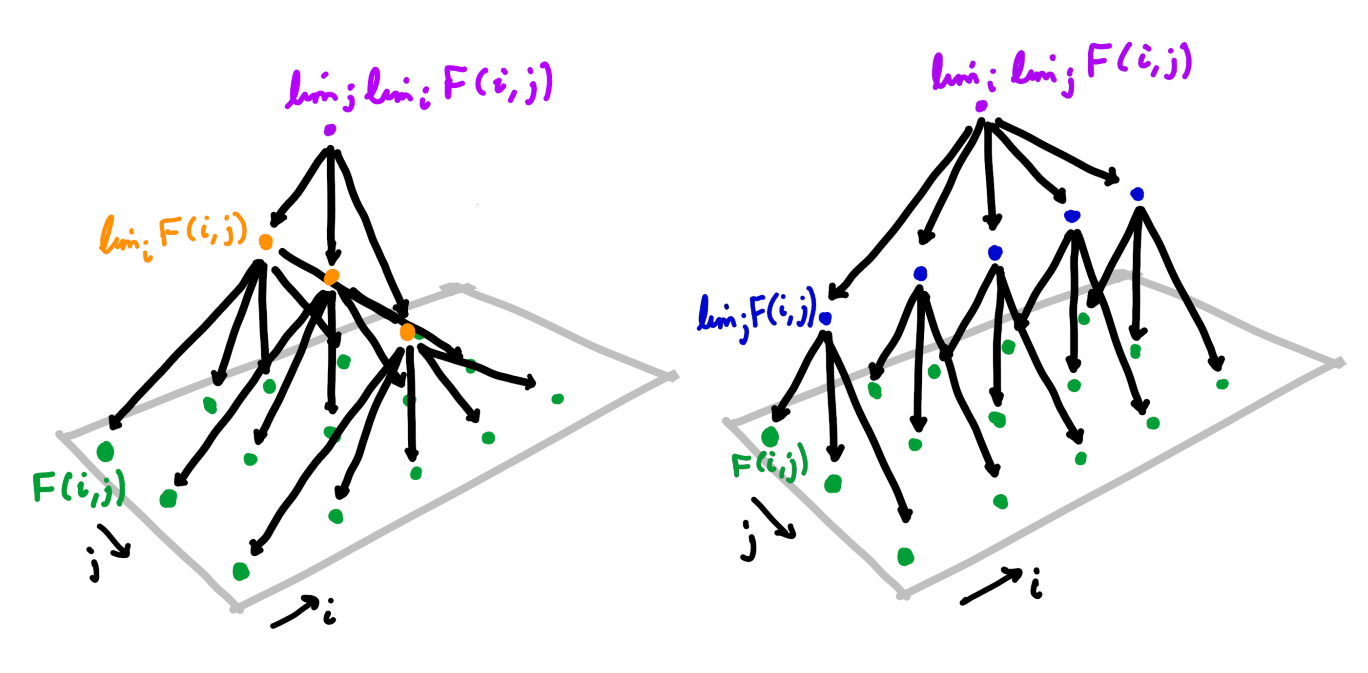A very useful fact in category theory is that limits commute with limits (and dually colimits commute with colimits). That is, given a functor $$F : I \times J \to C$$ we have $\lim_i \lim_j F(i, j) \cong \lim_j \lim_i F(i, j)$ under appropriate conditions.
In this post I will explain the precise statement of this theorem, and describe three proofs. The first proof directly uses universal properties. The second is a standard argument using the Yoneda embedding to reduce to the case of $$\text{Set}$$, which we will see is really the same proof in disguise. The third proof is an elegant argument using uniqueness of adjoints.